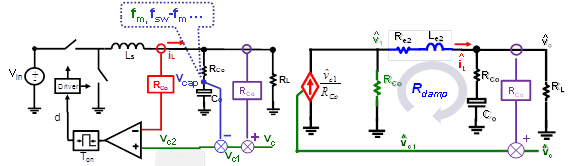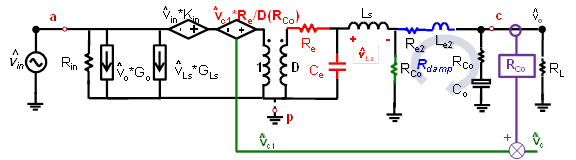Contact Us
LIBRARY

# Unified Equivalent Circuit Model of V2 ControlFig. 1. V2 Control with separated three feedback loop (a) and equivalent Circuit Model for control-port.
This nugget proposes a unified equivalent circuit model of V2 control. The direct output volt-age feedback in V2 control contains inductor current, capacitor voltage and load current information, which can be separated into 3 feedback loops, as shown in Fig.1(a) (use constant on-time as an example). Fig.1(b) shows that for constant on time structure, the inductor current feedback turns the circuit into a controlled current source while the capacitor voltage feedback turns this controlled current source into a controlled voltage source, which will be realized by a current source in parallel with a resistor. Moreover, due to the sideband frequency in the capacitor voltage feedback, the voltage source is a non-ideal source, with the non-idealness shown by a Re2-Le2 branch in Fig. 1(b), which forms a double pole at half of the switching frequency by resonating with the output capacitor. The damping of the double pole, which is shown as Rdamp in Fig.1(b), is related with the capacitor parameters and can be positive or negative. This explains why this structure is elegant when large ESR capacitors are employed (such as OSCON caps) while sub-harmonic oscillation occurs when low ESR capacitors are employed (such as ceramic caps). Fig. 2 shows a complete equivalent circuit model of V2 control, which is applicable to all kinds of V2 control. Generally speaking, the inductor current loop turns the circuit into a non-ideal current source, with the non-idealness shown by the Re-Ce branch, which will form a double pole by resonating with the power state inductor. The capacitor voltage loop turns the non-ideal current source into a non-ideal voltage source, with the non-idealness shown by the Re2-Le2 branch, which will form a double pole by resonating with power stage capacitor. The parameters of Re, Ce, Re2 and Le2 are different between different modulation schemes. This equivalent circuit model can be used to examine all the transfer functions (control-to-output, output impedance, input impedance and audio susceptibility) and can be extended very easily to a multi-phase structure and V2 control with a current ramp case.Fig. 2. Complete Equivalent Circuit Model for V2 Control.
INDUSTRY PARTNERS